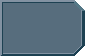## WARP SCALES

Discuss the site here - suggestions, comments, complaints, etc.

### WARP SCALES

I developed a lot easier formula to calculate the TNG warp speeds above of Warp 9.9. (then the formula in the article.)

Apart from that, the formula given in the article does not work for the canonical values and is always a bit off the mark.

Warp 9,9 - canon 3053 - formula in article 3065
Warp 9,99 - canon 7912 - formula in article 7900
Warp 9,9999 - canon 199516 - formular in article 199973

To my approach:

If you takes the given values and determines the exponents, a pattern will be noticed.

Warp 9,9 - 3053 -> exponent 3,5
Warp 9,99 - 7912 -> exponent 3,9
Warp 9,9999 - 199516 -> exponent 5,3

Subtract 3.3 each and multiplied by 10
0,2 - 2
0,6 - 6
2,0 - 20

If you now compare the number of 9s right the separator, this sequence of numbers exactly matches the formula n² + n.
1 - 2
2 - 6
3 - 12
4 - 20
5 - 30
6 - 42

You can even determine the missing exponent for 9.999 to 4.5. And thus the speed to 31609 xc.

So you can calculate the warp speed above 9.9 with:

xc = Wf ^ ( 3.3 + ( log(10-Wf)^2 ) / 10 - ( log(10-Wf) ) / 10 )

-log(10-Wf) gives exactly the number of 9s right the separator -> 10-9,99 = 0,01 and log(0,01) = -2

Lot easier and represents exactly the canonical values for 9.9; 9.99 and 9.9999.

Unfortunately, this formula does not work between 9 and 9.9.
Mark McWire
CrewmanPosts: 2
Joined: Tue Dec 01, 2015 10:03 pm

### Re: WARP SCALES

If you expand the exponent's formula by + 1 / ( 29 + (1 / (10-Wf) )^9 ), it will apply to all warp factors except 9.6. For 9.6 there will be 1979 instead of 1909.
Mark McWire
CrewmanPosts: 2
Joined: Tue Dec 01, 2015 10:03 pm